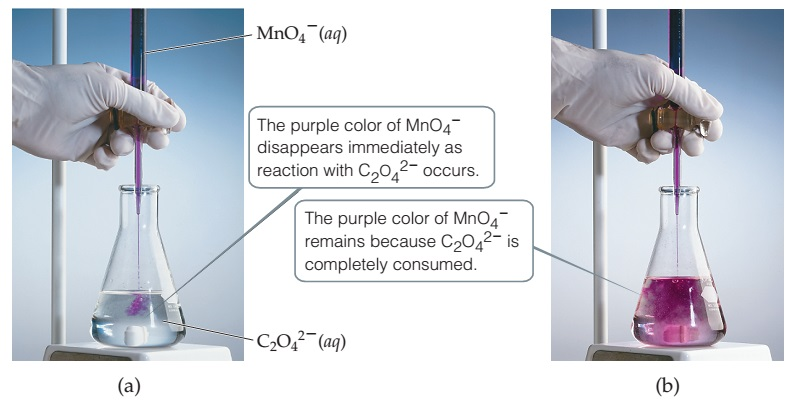# Problem: Titration of an acidic solution of Na2C2O4 with KMnO4(aq). The balanced chemical equation for this reaction is: 16 H+(aq) + 2 MnO4-(aq) + 5 C2O42-(aq)  →  2 Mn2+(aq) + 8 H2O(l) + 10 CO2(g) Which species is the reducing agent?

###### FREE Expert Solution
90% (223 ratings)
###### Problem Details

Titration of an acidic solution of Na2C2O4 with KMnO4(aq).The balanced chemical equation for this reaction is:

16 H+(aq) + 2 MnO4-(aq) + 5 C2O42-(aq)  →  2 Mn2+(aq) + 8 H2O(l) + 10 CO2(g)

Which species is the reducing agent?

Frequently Asked Questions

What scientific concept do you need to know in order to solve this problem?

Our tutors have indicated that to solve this problem you will need to apply the Redox Reactions concept. You can view video lessons to learn Redox Reactions. Or if you need more Redox Reactions practice, you can also practice Redox Reactions practice problems.